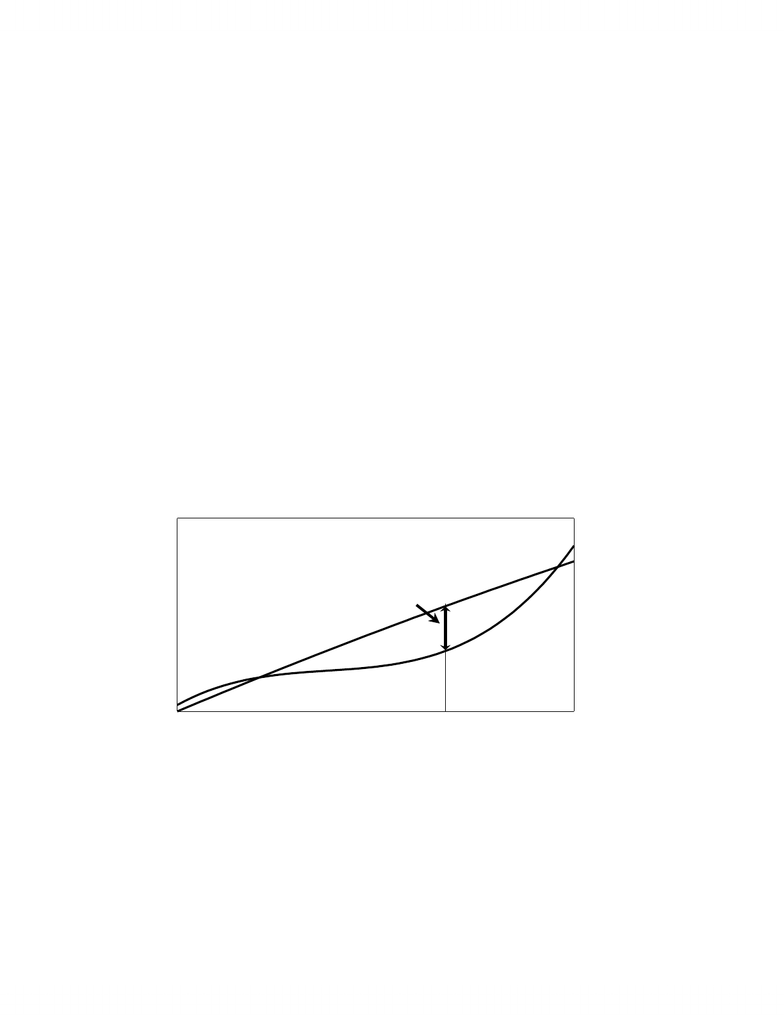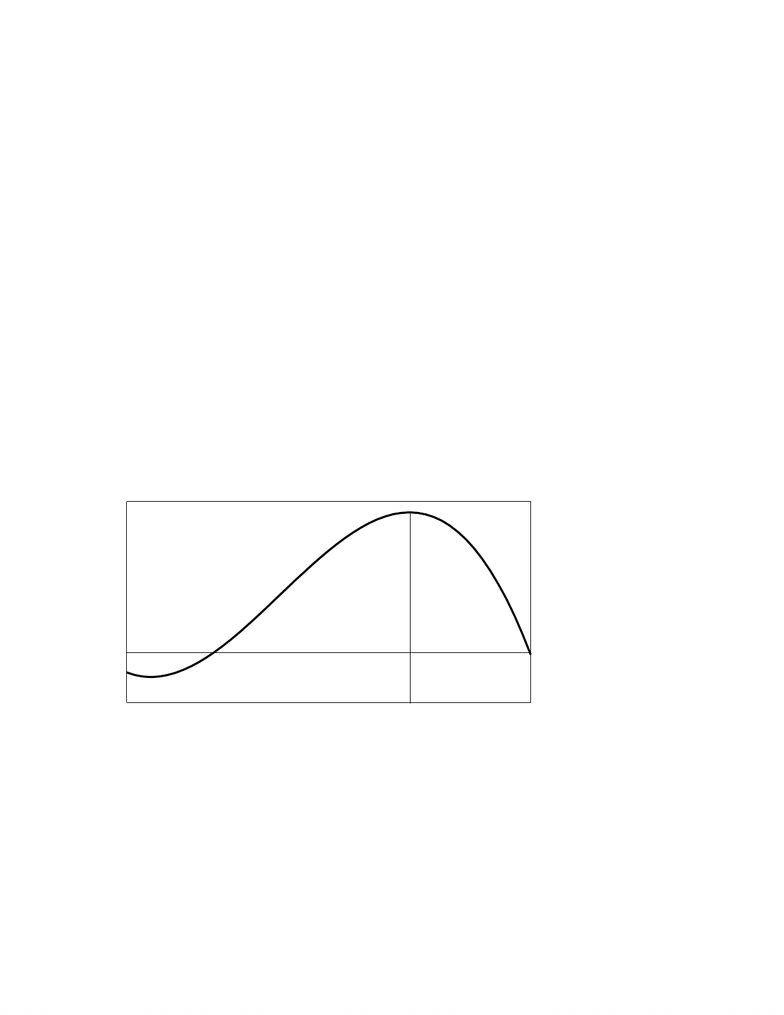Class Notes (1,100,000)
CA (630,000)
UTSG (50,000)
ECO (2,000)
A (10)
Lecture

ECO204Y1 Lecture Notes - Ceteris Paribus, Negative Number, Perfect Competition

Department
Economics
Course Code
ECO204Y1
Professor
A

This preview shows pages 1-3. to view the full 16 pages of the document.Principles of Economics: Short-run Firm Equilibrium
PROFIT MAXIMIZATION
Profit = Total Revenue (P*Q) – Total Costs
Accountants calculate Profit as operating Profit
= Total Revenue – Operating Costs
= Total Revenue – Variable Costs (in economic terminology)
(Operating Profit = Producer Surplus in economic terminology)
Accountants compare this operating profit with Capital to find the rate of return on capital
Economics calculate Economic Profit from all costs, including the cost of capital calculated
as the opportunity cost of Capital.
Definition: Economic Profit = Total Revenue – (Variable Costs + Fixed Costs)
Definition: Normal Profit = Opportunity Cost of Capital
0 Economic Profit = Normal Profit since it includes the Opportunity Cost of Capital
Economic Loss => Economic Profit < 0 (less than the Opportunity Cost of Capital)
Economic Profit => Economic Profit > 0 (greater than the Opportunity Cost of Capital)
Short-run Profit Maximization
=> Find the output that maximizes Profit (TR – TC)
=> (TR – TC)/q = 0 [d(TR – TC)/dq = 0]
=> TR/q – TC/q = 0 [dTR/dq - dTC/dq = 0]
=> MR = MC
[More formally:
Profit = TR – TC = PQ – (wL + rK) (w = wage rate and r = opportunity cost of Capital)
Profit Maximization => (PQ – wL – rK)/ Q = 0
P + QP/Q – wL/Q = 0
- 1 -

Only pages 1-3 are available for preview. Some parts have been intentionally blurred.Principles of Economics: Short-run Firm Equilibrium
P + QP/Q = MC since wL/Q = MC
MR = MC for all firms since P + QP/Q = MR]
Marginal Revenue equal to Marginal Cost gives the profit maximizing output but this
expression also includes the loss minimizing output if the price is below Average Cost. (There is
one exception to this rule as we will see).
E.g. Suppose that the following functions describe the Variable Cost and Fixed Cost of a
competitive firm VC = q3 – 59q2 + 1315q FC = 2000
This cubic function gives the correct shape for the Marginal Cost function but we will
eventually work only with more quadratic Variable Cost functions. Note that a change in quantity
changes Variable Cost but has no effect on Fixed Cost. Total Cost is the sum of both functions.
Suppose further that the firm’s Total Revenue function is TR = 1000q – 2q2.
TC = q
3
- 59q
2
+1315q +2000; TR = 1000q - 2q
2
0 10 20 30 40 50
Quantity
0
10
20
30
40
50
60 \$000
TC
TR
Max (TR - TC) => Max Profit
Slope TR = Slope TC => MR = MC
q*
Max Profit
Total Economic Profit is the difference between the Total Revenue and Total Cost functions
as shown in the diagram below. Total Economic Profit is greatest at the output where Total
Revenue – Total Cost is greatest. This occurs where the slopes of the two functions are equal, or
- 2 -

Only pages 1-3 are available for preview. Some parts have been intentionally blurred.Principles of Economics: Short-run Firm Equilibrium
when Marginal Revenue equals Marginal Cost since these are the slopes of Total Revenue and
Total Cost respectively.
The diagram below shows Economic Profit as a function of q for this firm.
Total Profit = 1000q - 2q
2
- (q
3
- 59q
2
+1315q +2000)
0 10 20 30 40 50
Quantity
0
5
10
15
-5
\$000
Total Profit (TR - TC)
q*
Max Profit
Comparison Marginal Revenue and Marginal Cost shows the maximum profit output more
clearly than comparison of Total Revenue and Total Cost.
Marginal Revenue = 1000 – 4q Marginal Cost = 3q2 - 118q + 1315
- 3 -
You're Reading a Preview

Unlock to view full version